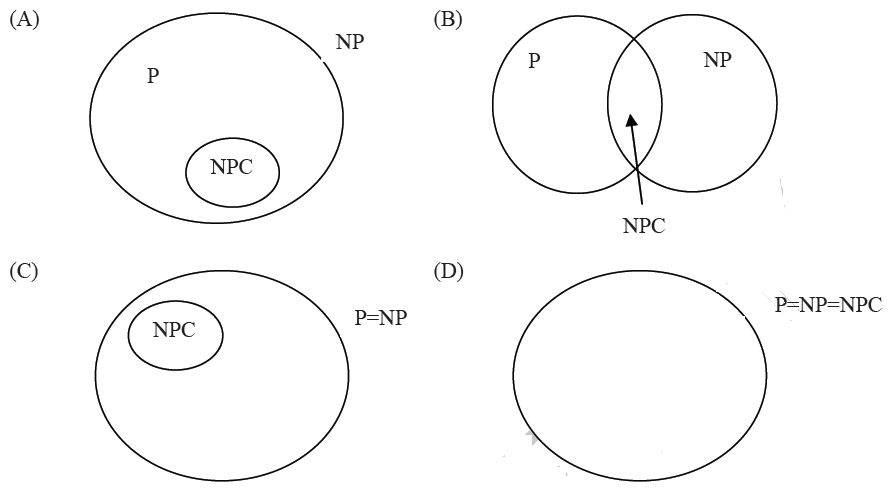GATE | GATE-CS-2014-(Set-1) | Question 48

• Last Updated : 28 Jun, 2021

Suppose a polynomial time algorithm is discovered that correctly computes the largest clique in a given graph. In this scenario, which one of the following represents the correct Venn diagram of the complexity classes P, NP and NP Complete (NPC)?(A) A
(B) B
(C) C
(D) D